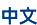## Numerical Linear Algebra

• 简介

Numerical linear algebra mainly studies theories and efficient algorithms related to matrix computations, including fast multiplications of matrices and vectors, direct and iterative solvers for large linear systems, designing preconditioners for linear equations, eigenvalues and  eigenvectors decomposition of matrices, least square methods, matrix functions computation, numerical precision and error analysis, etc. Numerical linear algebra is the core and foundation of computational mathematics. Many important numerical methods for differential equations, such as finite difference methods, finite element methods, spectral methods, boundary element methods, as well as regression and classification in big data and machine learning are often attributed to numerical linear algebra problems. One of the main purposes of developing high-performance computers is to solve super-large problems in numerical linear algebra.